Electric dipole moment function and line intensities for the ground state of carbon monxide*
Institute of Atomic and Molecular Physics, Sichuan University, Chengdu 610065, China
Science and Technology on Optical Radiation Laboratory, Beijing 100854, China

Corresponding author. E-mail: chengxl@scu.edu.cn

*Project supported by the National Natural Science Foundation of China (Grant Nos. 11374217 and 11474207).

Abstract

An accurate electric dipole moment function (EDMF) is obtained for the carbon monoxide (CO) molecule (X1Σ+) by fitting the experimental rovibrational transitional moments. Additionally, an accurate ab initio EDMF is found using the highly accurate, multi-reference averaged coupled-pair functional (ACPF) approach with the basis set, aug-cc-pV6Z, and a finite-field with ±0.005 a.u. (The unit a.u. is the abbreviation of atomic unit). This ab initio EDMF is very consistent with the fitted ones. The vibrational transition matrix moments and the Herman–Wallis factors, calculated with the Rydberg–Klein–Rees (RKR) potential and the fitted and ab initio EDMFs, are compared with experimental measurements. The consistency of these line intensities with the high-resolution transmission (HITRAN) molecular database demonstrates the improved accuracy of the fitted and ab initio EDMFs derived in this work.

PACS: 31.15.A–; 31.50.Bc; 33.20.Ea; 33.20.Vq
Keyword: electric dipole moment; vibrational transition matrix moments; Herman–Wallis factors; line intensities
1. Introduction

Carbon monoxide (CO) is a stable and important diatomic molecule and has been detected in interstellar clouds as well as the Earth’ s atmosphere. The spectral parameters of CO are included in well-known spectroscopic databases such as the high-resolution transmission (HITRAN) molecular database,  the high-temperature version of HITRAN called the HITEMP database,  and others. For CO and other molecules, calculations of line parameters are closely related to their electric dipole moment functions (EDMFs). With the progress of experimental technology, further investigation of the EDMF for CO (X1Σ + ) based on new experimental data is possible.

Several band systems of CO have been observed. Numerous spectral line parameter measurements of CO have been collected for the fundamental band 1– 0,  the first overtone 2– 0,  and the second overtone 3– 0.[8, 1217] Many studies in the past decade have focused on the 3– 0 band by using a variety of experimental designs. Chackerian and Valero measured the absolute rovibrational intensities of the 4– 0 band of carbon monoxide, and more recently Ogilvie et al. have evaluated the electric dipolar moments based on new experimental measurements of line strengths including the 4– 0 band of 12C16O. Furthermore, Chung et al. adapted a cavity ringdown spectral technique in the near-infrared region to measure the 5– 0 band for the first time.

Several EDMFs for the ground state of CO have been deduced with various methods based on experimental data with Δ υ ≤ 4.[8, 12, 19, 2126] For instance, based on the inversion of experimental intensity information, Chackerian and Tipping derived a hybrid EDMF in a Padé approximant. It was used to calculate the rovibrational intensities of the ground state CO (X1Σ + ) by Goorvitch et al.[27, 28, 29] In consideration of these pure vibrational matrix elements of the bands υ ′ – 0 with 0 ≤ υ ′ ≤ 5, Chung et al. derived an EDMF in a polynomial form. A considerable number of quantum chemical calculations of EDMFs for CO have been presented in various papers.[19, 3037] However, we find that the vibrational transition matrix elements (or rotationless transition matrix elements) calculated using the quantum chemical EDMFs along with the Rydberg– Klein– Rees (RKR) potential, or ab initio potential, actually disagree with the experimental results. Therefore, more accurate theoretical calculations are needed.

In this work, we adopt a direct fit approach to determine an EDMF for the CO X1Σ + state by utilizing individual, high-quality measurements for line intensities including the 5– 0 band. We also perform a series of theoretical calculations to determine the EDMF of the electronic state of CO (X1Σ + ). Based on the RKR potential, the eigenfunctions, Ψ υ , J(r), are determined by solving the radial Schrö dinger equation of nuclear motion. Eigenfunctions, Ψ υ , J(r), and EDMFs are used to determine the vibrational matrix elements and the Herman– Wallis factors for several bands of CO (X1Σ + ). Finally, we calculate the rovibrational line intensities of some bands of CO (X1Σ + ) by using the present fitted and ab initio EDMFs and compare them with the published experimental data.

2. Theoretical basis
2.1. Fitted EDMF method

The method of obtaining the EDMF experimentally, as developed by Tipping and Herman, is to assume a power series expansion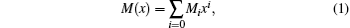and to fit the coefficient, Mi, to the measured rotationless transition matrix elements 〈 υ ′ , 0| M(r)| υ ″ , 0〉 . The single and double primes correspond to the upper and lower states, respectively. In Eq.  (1), x = (rre)/re, where re is the equilibrium separation and M0 represents the permanent dipole moment at the minimum potential where r = re. In a harmonic potential, M1x is related to the Δ υ = 1 transitions, while M1x2 is related to the Δ υ = 2 transitions and so on.

Using Eq.  (1), the rovibrational matrix elements can be written as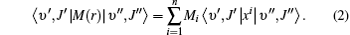Thus, the inclusion of rotational components allows a better determination of the Mi coefficients by fitting to a greater number of points.[39, 40] In order to derive the new EDMF from Eq.  (2), we identify the best available experimental results for each band. We use the results of Zou et al. for the fundamental band, Brulat et al.’ s for the first overtone band, Sung et al.’ s for the 3– 0 band, Chackerian et al.’ s for the 4– 0 band, and Chung et al.’ s for the 5– 0 band. Moreover, in order to obtain the accurate coefficient M0, the value of the matrix moment μ υ ′ = 0, υ ″ = 0 = 0.10982  Debye as reported by Chackerian et al. is also included in the fitting work. The weighted and unweighted fits of all data are performed.

2.2. Theoretical computational details

The ground state carbon monoxide molecule is a closed-shell molecule, and the electric configuration of the CO X1Σ + is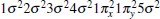, which has a1 symmetry. We compute the EDMF by using highly correlated ab initio methods, namely, the complete active space self-consistent field (CASSCF) approach followed by the highly accurate averaged coupled-pair functional (ACPF) method in the C2V point group.

The EDMF calculations are performed by using the MOLPRO 2009.1 program package,  with and without a finite-field approach with field values of ± 0.005  a.u. We separately use the aug-cc-pV6Z (aV6Z) and spdfghi aug-cc-pV6Z (spdfghi aV6Z) basis sets of Dunning et al. for both C and O atoms. These basis sets are sufficiently diffuse to describe long-range interactions so that a more accurate EDMF can be determined at larger internuclear separations. In this work, we only consider the electric correlation energy of valence electrons and only choose the full valence active spaces consisting of eight valence orbitals, which is denoted by (4220) in C2V point group symmetry.

With the aid of Le Roy’ s LEVEL 8.0 program,  the piecewise polynomial interpolation is performed by using numerical input potential energies and electric dipole moments. The rovibrational matrix elements are obtained through the use of the fitted EDMF and the theoretically calculated EDMF, together with an RKR potential energy function. The RKR potential curves for CO are constructed by using the purely numerical RKR method with Dunham coefficients from Velichko et al. who provided accurate molecular parameters for higher-lying vibrational levels (up to υ = 41).[49, 50]

3. Results and discussion
3.1. Details of calculations and results

The RKR potential is employed to calculate the transition wavenumbers, lower-state energies, and the expectation values 〈 υ ′ , J′ | xi| 0, J″ 〉 by using Le Roy’ s LEVEL 8.0 program. The rovibrational matrix elements are then evaluated from the measured line intensities according to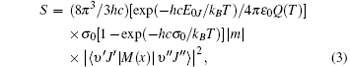where S is the line intensity for pure 12C16O in cm− 1/mol; h, c, and k are Planck constant, velocity of light, and Boltzmann constant, respectively; σ 0 is the transition wavenumber in cm− 1; T is the temperature; Q(T) is the total internal partition; m = 1/2[J′ (J′ + 1) − J″ (J″ + 1)] is a running number of values J″ + 1 for a line in branch R or − J″ for a line in branch P. A sequence of signs of the rovibrational matrix moments (+ − + − + + ) is adopted in a similar way to that in a previous study. Table  1 lists the rovibrational matrix elements derived from the experimental intensities and their experimental uncertainties. Finally, a fit of all listed rovibrational matrix elements to the system of linear Eq.  (2) is carried out by using a least-squares fit program. Six coefficients of the EDMF, M0, M1, M2, … , M5, are determined, and the polynomial equation of the weighted EDMF is written as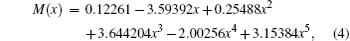whereas the unweighted EDMF equation is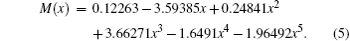Both equations implement an equilibrium separation of re = 1.12822  Å obtained from the constructed RKR potential. For comparison, the determined fitted EDMFs and those reported by Chung et al. are plotted against internuclear distance in Fig.  1. Meanwhile, the theoretical EDMFs calculated through using the ACPF method at the aV6Z set level with the field values of ± 0.005  a.u., those by Langhoff and Bauschlicher through using the aug-cc-pVQZ ACPF-field method, and those by Ogilvie et al. through using the MCSCF method are all plotted in Fig.  1.Table 1. Rovibrational transitional moments Mυ 0(m) of CO in units of Debye. The subscripts υ and 0 of Mυ 0(m) represent the υ -th upper vibrational level and the low vibrational level, respectively.

 Figure Option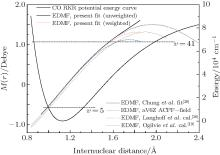Fig.  1. Electric dipole moment functions and RKR potential energy curve.

Within the respective valid range, the three fitted EDMFs are in close agreement with each other. According to the RKR potential, the classical turning points of the vibrational level υ = 5 are found to be approximately 0.967  Å and 1.322  Å . Therefore, the fitted EDMF is most reliable in the region between these two limits, which are slightly different from the results shown in Chung et al. (0.99  Å and 1.33  Å ). The valid range of the fitted EDMF is controlled by the largest overtones which are very sensitive to determining the higher coefficients of Mi in Eq.  (1). Thus, it is important to obtain experimental measurements for the largest possible values of υ ′ and Δ υ . The weighted and unweighted fitted EDMFs overlap together at 0.85  Å ≤ r ≤ 1.45  Å in the present work. In the valid range, all the fitted EDMFs agree well with the theoretical EDMF calculated using the ACPF method with a finite-field ± 0.005  a.u at the aV6Z basis set level (aV6Z ACPF-field). They also agree well with the theoretical results of Langhoff and Bauschlicher. The three lines outside the valid range are not consistent with the two theoretical EDMFs at large internuclear distances. Actually, the degree of agreement between the fitted EDMF of Chung et al. and the two theoretical EDMFs become continually worse when r ≥ 1.33  Å .

3.2. Theoretical results of EDMFs

According to geometrical optimization of the CO molecule, the equilibrium separation and the minimum energy are 1.1324  Å and − 113.197964  a.u. for the aV6Z basis set, and 1.1323  Å and − 113.198196  a.u for the spdfghi aV6Z basis set, respectively. These two values of re agree well with the experimental values of 1.1283  Å and 1.1282  Å obtained from the constructed RKR potential in the present work. The calculated energies and dipole moments each as a function of r are listed in Table  2 at the ACPF (4220) level using the aV6Z and spdfghi aV6Z basis sets. The two EDMFs almost converge both with and without using the finite-field approach. Here, we only compare the aV6Z ACPF-field EDMF with the three fitted and previous theoretical ones in Fig.  1.Table 2. Energies and electric dipole moments (EDMs) (a.u.)a for the X1Σ + state CO using the aug– cc– pV6Z and spdfgh aug– cc– pV6Z basis sets.

The aV6Z ACPF-field EDMF agrees well with the three fitted ones in their valid ranges. Compared with the theoretical results given by Langhoff and Bauschlicher,  the values of the dipole moments are smaller at small r and slightly larger at large r. The latest work of Oglivie et al. disagrees with the three fitted and the two theoretical ones.

4. Conclusions

By using a weighted and unweighted approach to fit the best available data of individual rovibrational transitions of CO (X1Σ + ), we obtain an accurate EDMF within the valid range of 0.967  Å ≤ r ≤ 1.322  Å , which agrees well with that of Chung et al. Meanwhile, an accurate ab initio EDMF is also calculated at the ACPF/aug-cc-pV6Z level with a finite-field of ± 0.005  a.u. The fitted EDMF in the valid range shows close agreement with the ab initio EDMF, and for small values of r, the electric dipole moment values are slightly smaller than the previous theoretical results of Langhoff and Bauschlicher. Combining the RKR potential curve and these EDMFs, the transition matrix moments are computed and compared with the experimental ones. The vibrational transition matrix moments μ υ ′ , υ calculated by using our unweighted fitted EDMF are in excellent agreement with the experimental values. The Herman– Wallis factors calculated using the present aV6Z ACPF-field EDMF also agree well with the experimental measurements. Comparisons are made among line intensities calculated here, reported in the HITRAN database and obtained from experimental measurements. The results clearly show that the present unweighted fitted and aV6Z ACPF-field EDMFs are accurate and useful in predicting the spectral properties of the CO molecule.

Reference